# Matrices

Library of functions operating on matrices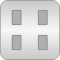# Package Contents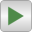Examples Examples demonstrating the usage of the Math.Matrices functions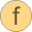toString Convert a matrix into its string representationisEqual Compare whether two Real matrices are identicalsolve Solve real system of linear equations A*x=b with a b vector (Gaussian elimination with partial pivoting)solve2 Solve real system of linear equations A*X=B with a B matrix (Gaussian elimination with partial pivoting)leastSquares Solve linear equation A*x = b (exactly if possible, or otherwise in a least square sense; A may be non-square and may be rank deficient)leastSquares2 Solve linear equation A*X = B (exactly if possible, or otherwise in a least square sense; A may be non-square and may be rank deficient)equalityLeastSquares Solve a linear equality constrained least squares problemLU LU decomposition of square or rectangular matrixLU_solve Solve real system of linear equations P*L*U*x=b with a b vector and an LU decomposition (from LU(..))LU_solve2 Solve real system of linear equations P*L*U*X=B with a B matrix and an LU decomposition (from LU(..))eigenValues Return eigenvalues and eigenvectors for a real, nonsymmetric matrix in a Real representationeigenValueMatrix Return real valued block diagonal matrix J of eigenvalues of matrix A (A=V*J*Vinv)singularValues Return singular values and left and right singular vectorsQR Return the QR decomposition of a square matrix with optional column pivoting (A(:,p) = Q*R)hessenberg Return upper Hessenberg form of a matrixrealSchur Return the real Schur form (rsf) S of a square matrix A, A=QZ*S*QZ'cholesky Return the Cholesky factorization of a symmetric positive definite matrixbalance Return a balanced form of matrix A to improve the condition of AbalanceABC Return a balanced form of a system [A,B;C,0] to improve its condition by a state transformationtrace Return the trace of matrix A, i.e., the sum of the diagonal elementsdet Return determinant of a matrix (computed by LU decomposition; try to avoid det(..))inv Return inverse of a matrix (try to avoid inv(..))rank Return rank of a rectangular matrix (computed with singular values)conditionNumber Return the condition number norm(A)*norm(inv(A)) of a matrix Arcond Return the reciprocal condition number of a matrixnorm Return the p-norm of a matrixfrobeniusNorm Return the Frobenius norm of a matrixnullSpace Return the orthonormal nullspace of a matrixexp Return the exponential of a matrix by adaptive Taylor series expansion with scaling and balancingintegralExp Return the exponential and the integral of the exponential of a matrixintegralExpT Return the exponential, the integral of the exponential, and time-weighted integral of the exponential of a matrixcontinuousLyapunov Return solution X of the continuous-time Lyapunov equation X*A + A'*X = CcontinuousSylvester Return solution X of the continuous-time Sylvester equation A*X + X*B = CcontinuousRiccati Return solution X of the continuous-time algebraic Riccati equation A'*X + X*A - X*B*inv(R)*B'*X + Q = 0 (care)discreteLyapunov Return solution X of the discrete-time Lyapunov equation A'*X*A + sgn*X = CdiscreteSylvester Return solution of the discrete-time Sylvester equation A*X*B + sgn*X = CdiscreteRiccati Return solution of discrete-time algebraic Riccati equation A'*X*A - X - A'*X*B*inv(R + B'*X*B)*B'*X*A + Q = 0 (dare)sort Sort the rows or columns of a matrix in ascending or descending orderflipLeftRight Flip the columns of a matrix in left/right directionflipUpDown Flip the rows of a matrix in up/down direction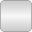LAPACK Interface to LAPACK library (should usually not directly be used but only indirectly via Modelica.Math.Matrices)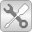Utilities Utility functions that should not be directly utilized by the user

# Information

This information is part of the Modelica Standard Library maintained by the Modelica Association.

#### Library content

This library provides functions operating on matrices. Below, the functions are ordered according to categories and a typical call of the respective function is shown. Most functions are solely an interface to the external LAPACK library.

Note: A' is a short hand notation of transpose(A):

Basic Information

• toString(A) - returns the string representation of matrix A.
• isEqual(M1, M2) - returns true if matrices M1 and M2 have the same size and the same elements.

Linear Equations

• solve(A,b) - returns solution x of the linear equation A*x=b (where b is a vector, and A is a square matrix that must be regular).
• solve2(A,B) - returns solution X of the linear equation A*X=B (where B is a matrix, and A is a square matrix that must be regular)
• leastSquares(A,b) - returns solution x of the linear equation A*x=b in a least squares sense (where b is a vector and A may be non-square and may be rank deficient)
• leastSquares2(A,B) - returns solution X of the linear equation A*X=B in a least squares sense (where B is a matrix and A may be non-square and may be rank deficient)
• equalityLeastSquares(A,a,B,b) - returns solution x of a linear equality constrained least squares problem: min|A*x-a|^2 subject to B*x=b
• (LU,p,info) = LU(A) - returns the LU decomposition with row pivoting of a rectangular matrix A.
• LU_solve(LU,p,b) - returns solution x of the linear equation L*U*x[p]=b with a b vector and an LU decomposition from "LU(..)".
• LU_solve2(LU,p,B) - returns solution X of the linear equation L*U*X[p,:]=B with a B matrix and an LU decomposition from "LU(..)".

Matrix Factorizations

• (eval,evec) = eigenValues(A) - returns eigen values "eval" and eigen vectors "evec" for a real, nonsymmetric matrix A in a Real representation.
• eigenValueMatrix(eval) - returns real valued block diagonal matrix of the eigenvalues "eval" of matrix A.
• (sigma,U,VT) = singularValues(A) - returns singular values "sigma" and left and right singular vectors U and VT of a rectangular matrix A.
• (Q,R,p) = QR(A) - returns the QR decomposition with column pivoting of a rectangular matrix A such that Q*R = A[:,p].
• (H,U) = hessenberg(A) - returns the upper Hessenberg form H and the orthogonal transformation matrix U of a square matrix A such that H = U'*A*U.
• realSchur(A) - returns the real Schur form of a square matrix A.
• cholesky(A) - returns the cholesky factor H of a real symmetric positive definite matrix A so that A = H'*H.
• (D,Aimproved) = balance(A) - returns an improved form Aimproved of a square matrix A that has a smaller condition as A, with Aimproved = inv(diagonal(D))*A*diagonal(D).

Matrix Properties

• trace(A) - returns the trace of square matrix A, i.e., the sum of the diagonal elements.
• det(A) - returns the determinant of square matrix A (using LU decomposition; try to avoid det(..))
• inv(A) - returns the inverse of square matrix A (try to avoid, use instead "solve2(..) with B=identity(..))
• rank(A) - returns the rank of square matrix A (computed with singular value decomposition)
• conditionNumber(A) - returns the condition number norm(A)*norm(inv(A)) of a square matrix A in the range 1..∞.
• rcond(A) - returns the reciprocal condition number 1/conditionNumber(A) of a square matrix A in the range 0..1.
• norm(A) - returns the 1-, 2-, or infinity-norm of matrix A.
• frobeniusNorm(A) - returns the Frobenius norm of matrix A.
• nullSpace(A) - returns the null space of matrix A.

Matrix Exponentials

• exp(A) - returns the exponential e^A of a matrix A by adaptive Taylor series expansion with scaling and balancing
• (phi, gamma) = integralExp(A,B) - returns the exponential phi=e^A and the integral gamma=integral(exp(A*t)*dt)*B as needed for a discretized system with zero order hold.
• (phi, gamma, gamma1) = integralExpT(A,B) - returns the exponential phi=e^A, the integral gamma=integral(exp(A*t)*dt)*B, and the time-weighted integral gamma1 = integral((T-t)*exp(A*t)*dt)*B as needed for a discretized system with first order hold.

Matrix Equations

• continuousLyapunov(A,C) - returns solution X of the continuous-time Lyapunov equation X*A + A'*X = C
• continuousSylvester(A,B,C) - returns solution X of the continuous-time Sylvester equation A*X + X*B = C
• continuousRiccati(A,B,R,Q) - returns solution X of the continuous-time algebraic Riccati equation A'*X + X*A - X*B*inv(R)*B'*X + Q = 0
• discreteLyapunov(A,C) - returns solution X of the discrete-time Lyapunov equation A'*X*A + sgn*X = C
• discreteSylvester(A,B,C) - returns solution X of the discrete-time Sylvester equation A*X*B + sgn*X = C
• discreteRiccati(A,B,R,Q) - returns solution X of the discrete-time algebraic Riccati equation A'*X*A - X - A'*X*B*inv(R + B'*X*B)*B'*X*A + Q = 0

Matrix Manipulation

• sort(M) - returns the sorted rows or columns of matrix M in ascending or descending order.
• flipLeftRight(M) - returns matrix M so that the columns of M are flipped in left/right direction.
• flipUpDown(M) - returns matrix M so that the rows of M are flipped in up/down direction.